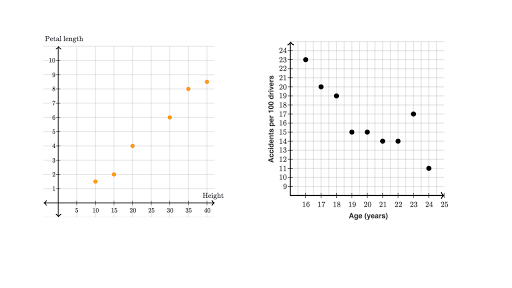# Exploring Bivariate Numerical Data Assessment Test

10 Questions | Total Attempts: 129SettingsFrom the exploration of linear regression to the assessment of the strength of linear models, take this assessment test to evaluate your knowledge of how to explore bivariate numerical data.

• 1.
Data on which each of two variables, where each value of one of the variables is paired with a value of the other variable is called...
• A.

Clear data

• B.

Bivariate data

• C.

Real data

• D.

Three diagram

• 2.
Which of these is used to represent a bivariate data?
• A.

Picture

• B.

Scatter plot

• C.

Lifeline

• D.

Slope

• 3.
What can be used to model the relationship between quantitative variables and make predictions?
• A.

Regression

• B.

Analysis

• C.

Line

• D.

Table

• 4.
A type of table in a matrix format that displays the frequency distribution variables is...
• A.

Curvilinear table

• B.

Graphical table

• C.

Contingency table

• D.

Plotting matrix table

• 5.
A table in which each variable has only two levels is called...
• A.

1x1

• B.

1x2

• C.

2x1

• D.

2x2

• 6.
A statistical test applied to sets of categorical data to evaluate how likely it is that any observed difference between the sets arose by chance is called...
• A.

Pearson's chi-squared test

• B.

Litmus test

• C.

Regression test

• D.

Analysis

• 7.
A test that examines the association of two categorical variables is...
• A.

Ion Dic's test

• B.

Barnard's test

• C.

Cuper's test

• D.

LifAton's test

• 8.
What kind of test is used to examine the significance of the contingency between two kinds of classification?
• A.

Fisher's exact test

• B.

Plot test

• C.

Scatter test

• D.

Round test

• 9.
The rows in a contingency table are referred to as...
• A.

Lines

• B.

Plots

• C.

Slopes

• D.

Stubs

• 10.
The columns in a contingency table are referred to as...
• A.

Slopes

• B.

Cuts

• C.

Lines

• D.

Rows

Related TopicsBack to top